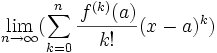## Saturday, March 11, 2006

### Taylor Series

The Taylor Series is one of the most useful results in all of mathematics. The method in all its generality was first presented by Brook Taylor. To see an application for Taylor's Series, check out Euler's Formula.

The proof for Taylor's Series depends on basic calculus.

For anyone who does not feel comfortable with the notation f(x) or the concept of a mathematical function, start here.

For anyone who does not know about derivatives or about the notation f'(x), f''(x), or fn(x), start here.

Theorem: Taylor Series
if
(a) f is a function with derivatives of all orders
(b) (lim n → inf)[f(n+1)(z)]/(n+1)!(x-a)n+1 = 0
then:
f(x) = f(a) + f'(a)(x-a) + [f''(a)/2!](x-a)2 + .... + [f(n)(a)/n!](x-a)n + ....

Proof:

(1) Let n be an arbitrary positive integer.

(2) From Taylor's Formula, we have:
f(x) = Pn(x) + Rn(x)

where:

Pn(x) = f(a) + f'(a)(x-a) + f''(a)[(x-a)2]/2! + ... + f(n)[(x-a)n]/n!

and

Rn(x) = [f(n+1)(z)]/(n+1)!(x-a)n+1

where z is between a and x.

(3) From assumption b, we see that:
lim (n → inf) Rn(x) = 0.

(4) Then it follows that:
f(x) = lim (n → inf) Pn(x) == f(a) + f'(a)(x-a) + [f''(a)/2!](x-a)2 + .... + [f(n)(a)/n!](x-a)n + ....

QED

References